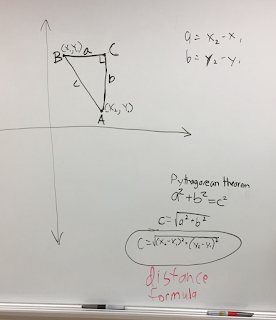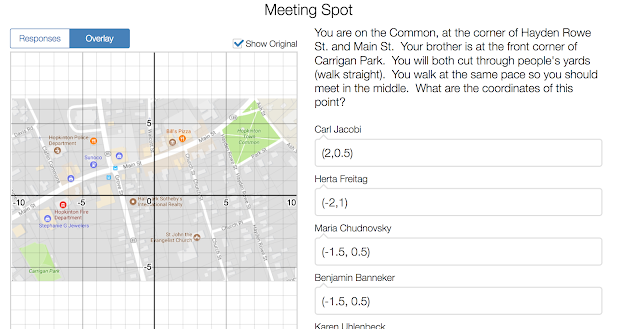Thursday, September 7, 2017

Geometry #VNPS Desmos Day 4

Day 3 in Geometry was a summer assessment on a summer packet.

Day 4 we moved into learning about the Distance Formula and the Midpoint Formula.  Kids "know" the distance formula.  They have used it.  They may forget what is added or subtracted, but it comes back to them.  I wanted them to see where it came from, so I had them up at the boards #VNPS and walked them through these instructions to graph on a coordinate plane, work their way to the Pythagorean Theorem (which I let them try to spell out and most of the students thought it was the "PythagoreaM TheorM" - so we corrected that misunderstanding.)  I had them solve it for C and replace with x s and ys.  They finally saw the connection between the Distance Formula and the Pythagorean Theorem!

Here is a picture of group work:Then, I made a Desmos activity that started with some thoughts on the Distance Formula and the Pythagorean Theorem.  It moves into asking them to figure out where the Midpoint Formula comes from.  Next, I brought a map of our town, Hopkinton, into a coordinate plane and asked them to find a distance.  Then, I did the normal "If somebody walks this way around the corner and somebody takes a shortcut, how much does the shortcut save?"  It took a lot longer than I thought.  I purposely did not put the fire station on a nice integer point, so they were struggling to figure out what decimals to use and then oh my, having to work through the distance formula with decimals was tough.  But, they did understand that they could have used the Pythagorean Theorem or the Distance Formula.  Most opted for the Distance Formula.  Then, I had them find a midpoint and that was a little easier.

One question I asked that I liked was about the Distance Formula and "Name two mistakes a student might make when using this Distance Formula."  Here is a snapshot of some results:Here is a picture of my Desmos Hopkinton Map slide:Meeting Spot: Midpoint: